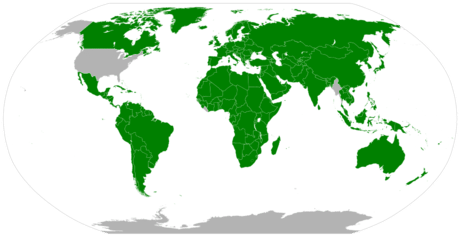# 1.6: SI Base Units

$$\newcommand{\vecs}{\overset { \rightharpoonup} {\mathbf{#1}} }$$ $$\newcommand{\vecd}{\overset{-\!-\!\rightharpoonup}{\vphantom{a}\smash {#1}}}$$$$\newcommand{\id}{\mathrm{id}}$$ $$\newcommand{\Span}{\mathrm{span}}$$ $$\newcommand{\kernel}{\mathrm{null}\,}$$ $$\newcommand{\range}{\mathrm{range}\,}$$ $$\newcommand{\RealPart}{\mathrm{Re}}$$ $$\newcommand{\ImaginaryPart}{\mathrm{Im}}$$ $$\newcommand{\Argument}{\mathrm{Arg}}$$ $$\newcommand{\norm}{\| #1 \|}$$ $$\newcommand{\inner}{\langle #1, #2 \rangle}$$ $$\newcommand{\Span}{\mathrm{span}}$$ $$\newcommand{\id}{\mathrm{id}}$$ $$\newcommand{\Span}{\mathrm{span}}$$ $$\newcommand{\kernel}{\mathrm{null}\,}$$ $$\newcommand{\range}{\mathrm{range}\,}$$ $$\newcommand{\RealPart}{\mathrm{Re}}$$ $$\newcommand{\ImaginaryPart}{\mathrm{Im}}$$ $$\newcommand{\Argument}{\mathrm{Arg}}$$ $$\newcommand{\norm}{\| #1 \|}$$ $$\newcommand{\inner}{\langle #1, #2 \rangle}$$ $$\newcommand{\Span}{\mathrm{span}}$$$$\newcommand{\AA}{\unicode[.8,0]{x212B}}$$

### How long is a yard?

It depends on whom you ask, and when you asked the question. Today we have a standard definition of the yard, which you can see marked on every football field. If a football player moves the ball ten yards, they get a first down, and it doesn't matter whether they are playing in Los Angeles, Dallas, or Green Bay. At one time, however, that yard was arbitrarily defined as the distance from the tip of the king's nose to the end of his outstretched hand. Of course, the problem in that case is simple: new king, new distance (and then you have to re-mark all those football fields).

## SI Base Units

All measurements depend on the use of units that are well known and understood. The English system of measurement units (inches, feet, ounces, etc.) are not used in science because of the difficulty in converting from one unit to another. The metric system is used because all metric units are based on multiples of 10, making conversions very simple. The metric system was originally established in France in 1795. The International System of Units is a system of measurement based on the metric system. The acronym SI is commonly used to refer to this system and stands for the French term, Le Système International d'Unités. The SI was adopted by international agreement in 1960 and is composed of seven base units, listed in Table $$\PageIndex{1}$$.

Table $$\PageIndex{1}$$: SI Base Units of Measurement
Quantity SI Base Unit Symbol
Length meter $$\text{m}$$
Mass kilogram $$\text{kg}$$
Temperature kelvin $$\text{K}$$
Time second $$\text{s}$$
Amount of a Substance mole $$\text{mol}$$
Electric Current ampere $$\text{A}$$
Luminous Intensity candela $$\text{cd}$$

The first units are frequently encountered in chemistry. All other measurement quantities, such as volume, force, and energy, can be derived from these seven base units.Figure $$\PageIndex{1}$$: Meter Standard. (Credit: Courtesy of US Department of Commerce; Courtesy of National Institute of Standards and Technology; Source: http://commons.wikimedia.org/wiki/File:US_National_Length_Meter.JPG(opens in new window); http://commons.wikimedia.org/wiki/File:Standard_kilogram,_2.jpg(opens in new window); License: Public Domain)Figure $$\PageIndex{2}$$: Kilogram standard (Credit: Courtesy of National Institute of Standards and Technology; Source: http://commons.wikimedia.org/wiki/File:Standard_kilogram,_2.jpg(opens in new window); License: Public Domain)

The map below shows the adoption of the SI units in countries around the world. The United States has legally adopted the metric system for measurements, but does not use it in everyday practice. Great Britain and much of Canada use a combination of metric and imperial units.Figure $$\PageIndex{3}$$: Areas of the world that use the metric system (green). (Credit: User:Canuckguy/Wikipedia and User:AzaToth/Wikimedia Commons; Source: http://commons.wikimedia.org/wiki/File:Metric_system_adoption_map.svg(opens in new window); License: Public Domain)

## Summary

• The SI system is based on multiples of ten.
• There are seven basic units in the SI system.
• Five of these seven SI units are commonly used in chemistry.

## Review

1. What does SI stand for?